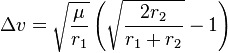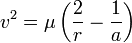# Hohmann transfer orbits (delta-v)

• louischaman
In summary, the equation for delta v is delta v = v2 - v1. I am trying to find the equation for v2 and v1 but I am getting stuck.

#### louischaman

I am writing an essay on Space travel for my A level coursework. I am writing about Hohmann transfer orbits.
http://en.wikipedia.org/wiki/Hohmann_transfer_orbit

It explains how you can derive this:From this:I can't do the in between steps.
help would be appreciated

I don't fully understand this either, and would like to know :P

However! I think the process would be;

v1² = u (2/r1 - 1/a )
v2² = u (2/r2 - 1/a )

with v1 v2 r1 r2 correponding to a velocity of X when you're in X orbit.

Anyway, i tried minusing them, and got into trouble :X, anyone help?

Well, consider delta v: delta v = v2 - v1.

Square that: (delta v)^2 = v2^2 - 2v2v1 + v1^2.

Now, from the original equation, v2 = sqrt(mu)sqrt(2/r2 - 1/a)
and v1 = sqrt(mu)sqrt(2/r1 - 1/a)
so -2v2v1 = -mu sqrt((2/r2 - 1/a)(2/r1 - 1/a))
and v2^2 = mu(2/r2 - 1/a)
and v1^2 = mu(2/r1 - 1/a)

so (delta v)^2 = mu(2/r2 - 1/a) - mu sqrt((2/r2 - 1/a)(2/r1 - 1/a)) + mu(2/r1 - 1/a)

Some algebra should get you to something that looks like the right side of the target equation. You'll probably need some tricks (partial fractions and similar) to force it into the right form.

]Hey there, thanks for the tip, however (and this is more maths related i guess) I'm having trouble getting there;

I've got to

delta v² = 2u [ 1/r1 + 1/r2 - 2/2a - sqrt(4/r1r2 - 2/r1a - 2/r2a + 1/a²)]

Really stuck on what to do next, I know what the answer is, but i can't seem to get rid of the a'sany help would be appreciated, and yeah i need to learn how to use the proper formula thingy rather than just typing it out, any help there would be great too :P is it a separate program because the latex thing in advanced is useless

Last edited:
$$\Delta$$v² = 2 $$\mu$$ [ $$\frac{1}{r1}$$ + $$\frac{1}{r2}$$ - $$\frac{2}{2a}$$ - $$\sqrt{\frac{4}{r1r2}$$-$$\frac{2}{r1a}$$-$$\frac{2}{r2a}$$+$$\frac{1}{a^2}$$

excuse my failure with latex, all of the last bit is suposed to be square rooted...however i can't work that out :P

I can't get any further from hereHey, sorry for the bump but this is a really annoying problem =[